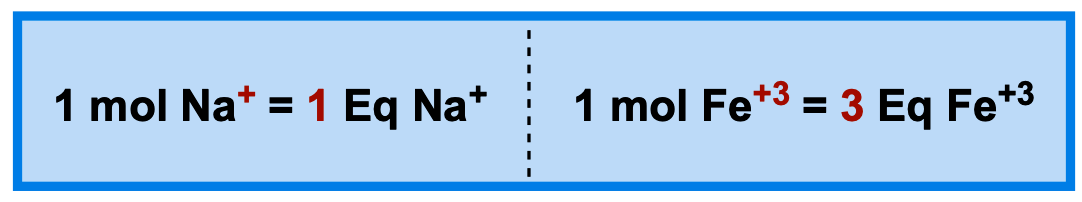Clutch Prep is now a part of Pearson

# Equivalents

See all sections
Sections
Solutions
Solubility and Intermolecular Forces
Solutions: Mass Percent
Percent Concentrations
Molarity
Osmolarity
Parts per Million (ppm)
Solubility: Temperature Effect
Intro to Henry's Law
Henry's Law Calculations
Dilutions
Solution Stoichiometry
Electrolytes (Simplified)
Equivalents
Molality
The Colligative Properties
Boiling Point Elevation
Freezing Point Depression
Osmosis
Osmotic Pressure

Equivalents are used to measure individual ion amount present in body fluids and intravenous solutions.

###### Equivalents (Eq)

Concept #1: Equivalent equals to 1 mol of positive or negative charge.Example #1: Calculate number of Equivalents in each of the following:

a) 1 mole of Ca2+ b) 2 moles of PO43-

Concept #2: Normality

Example #2: Calculate the Normality of 0.35 mole of Mg2+ ions present in a 300 mL of blood.

Practice: Calculate mass (grams) needed for the following ion equivalent: 1.5 mEq of Na+ ions.

Practice: The concentration of Cl- ion in blood is approximately 105 mEq/L. How many milliliters of blood would be needed to obtain 1.4 g of Cl- ions?

Practice: An intravenous saline solution contains 140 mEq/L of Na+. How many mEq of Na+ are present in 750 mL of the solution?

Practice: Calculate the normality (mEq/L) of potassium ions in a 500 mL Ringer’s solution that is 2.0 x 10-3 M in potassium ions.